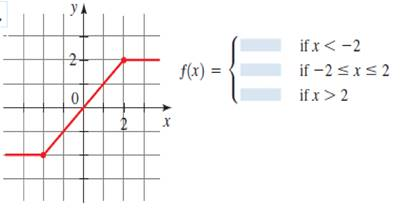# The formula of the curve.### Precalculus: Mathematics for Calcu...

6th Edition
Stewart + 5 others
Publisher: Cengage Learning
ISBN: 9780840068071### Precalculus: Mathematics for Calcu...

6th Edition
Stewart + 5 others
Publisher: Cengage Learning
ISBN: 9780840068071

#### Solutions

Chapter 2.2, Problem 49E
To determine

## To find : The formula of the curve.

Expert Solution

f(x)={2     if  x<2x       if 2x22       if x>2

### Explanation of Solution

Given information :Formula used :

The equation of the straight line parallel to X-axis can be written as y=a , where a is the Y-coordinate of any point lie on that line.

The equation of the straight line can be written as y=mx+c , where m is the gradient of the line and c is the Y intercept.

Calculation :

The graph right side of the x=2 is a line parallel to X-axis.

It is clear that all the Y coordinates of the points lie on that line is 2 . Therefore, the equation of the that line is y=2

The graph left side of the x=2 is a line parallel to X-axis.

It is clear that all the Y coordinates of the points lie on that line is 2 . Therefore, the equation of the that line is y=2 .

The graph between x=2 and x=2 is a straight line .

It passes through the origin. Hence, c=0

Coordinate of a point lies on that line is (2,2)

Since, it passes through the origin, m=22=1

Therefore, the formula of the curve is y=x

If we combine three equation and take y=f(x) , it can be written as

f(x)={2     if  x<2x       if 2x22       if x>2

### Have a homework question?

Subscribe to bartleby learn! Ask subject matter experts 30 homework questions each month. Plus, you’ll have access to millions of step-by-step textbook answers!